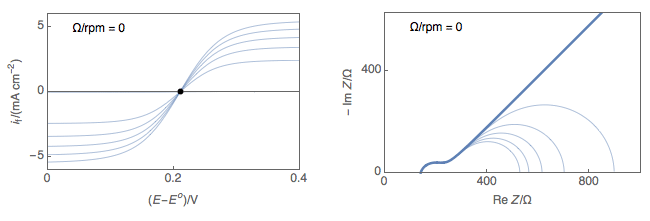Topic 5 min read# The easy way to D – the diffusion coefficient

Latest updated: February 2, 2022

Performing Electrochemical Impedance Spectroscopy (EIS) experiments on a Rotating Disk Electrode (RDE) at Open Circuit Potential (OCP) is an easy way to measure the diffusion coefficients of the electroactive species in the considered electrolyte, assuming that both redox species have the same diffusion coefficients.

On an RDE, the Faradaic current depends on the electron transfer rate and the concentration of species reaching the interface. The concentration itself depends on the mass transport conditions of the experiment: i) the convection ie the rotation rate of the electrode; ii) the diffusion ie the diffusion coefficient of the electroactive species. Fitting impedance data obtained on an RDE allows users to access the diffusion coefficient $D$ of both redox species, assuming they are the same.

Bounded diffusion impedance element $\mathrm{W}_{\delta}$ measurements at several rotation rates are required, as well as fitting and post-fitting processing of the data.

The analytical approximation of the bounded diffusion impedance, represented by the element $\mathrm{W}_{\mathrm{inf}}$ allows a direct determination of $D$ with only one single measurement at one rotation speed and fitting analysis on one single data set.

Please see Application Note 66 for further details on how to perform such an analysis using Z Fit in EC-Lab®.

Figure 1 shows typical Nyquist representations of a redox reaction occurring on an RDE at various rotations rates. At 0 rpm, interfacial concentrations depend on diffusion only, which is considered semi-infinite and the only stationary point is at OCP. At non-zero rotation rates any point on the I vs. E curve can be considered stationary.Figure 1: left) $I vs. E$ curves and right) Nyquist impedance diagram representations
of a redox reaction occurring on an RDE at various rotations rates.

Rotating Disk Electrode RDE diffusion coefficient EIS bounded diffusion potentiostat

## Related products

Work smarter. Not harder.

Tech-tips, theory, latest functionality, new products & more.

No thanks!